Ex 3.3

Chapter 3 Class 10 Pair of Linear Equations in Two Variables
Serial order wise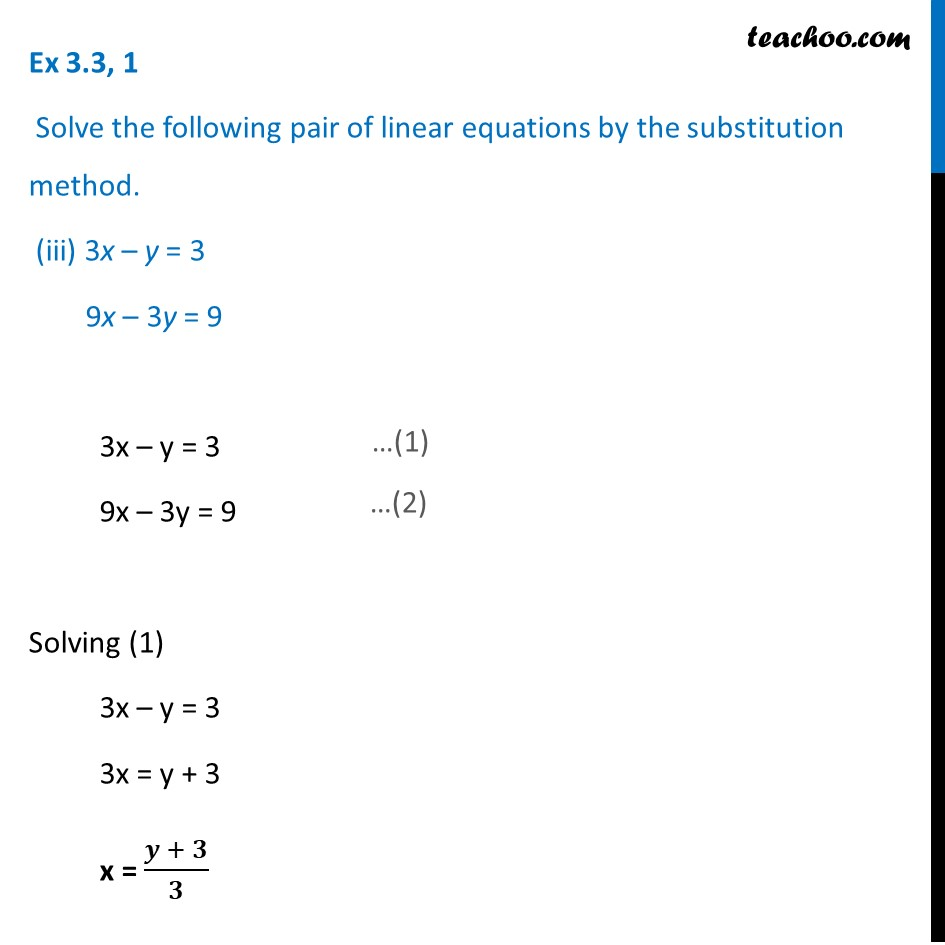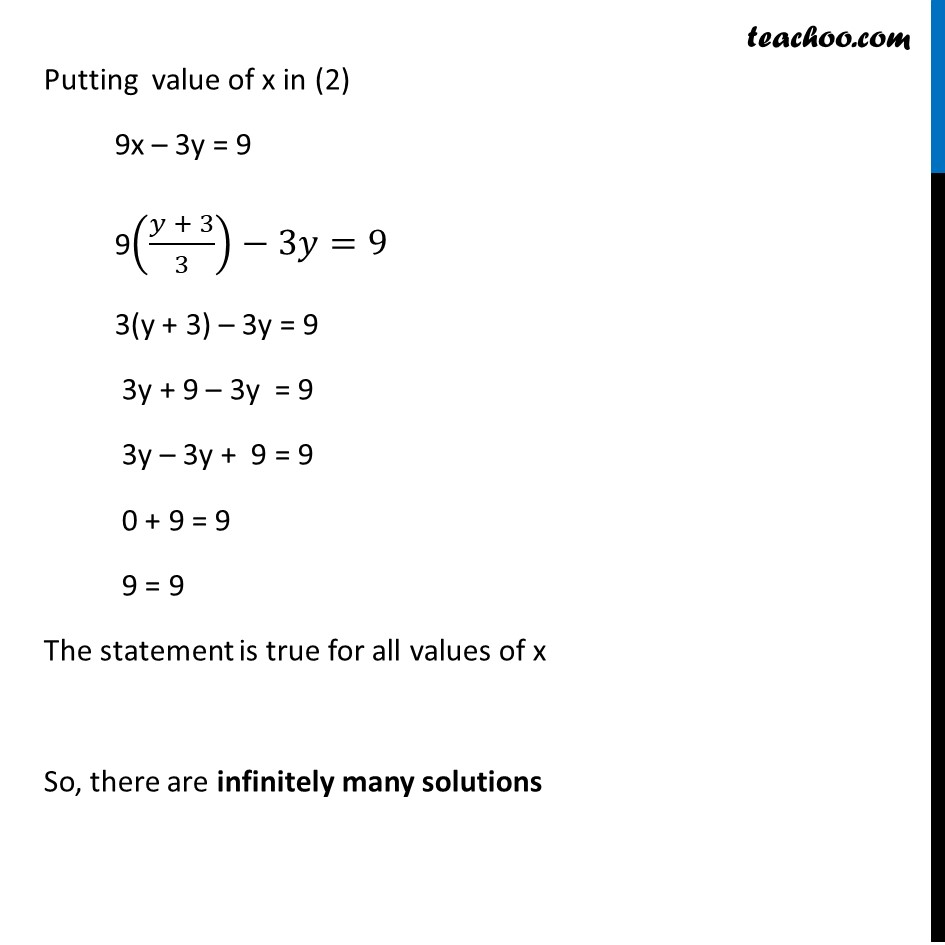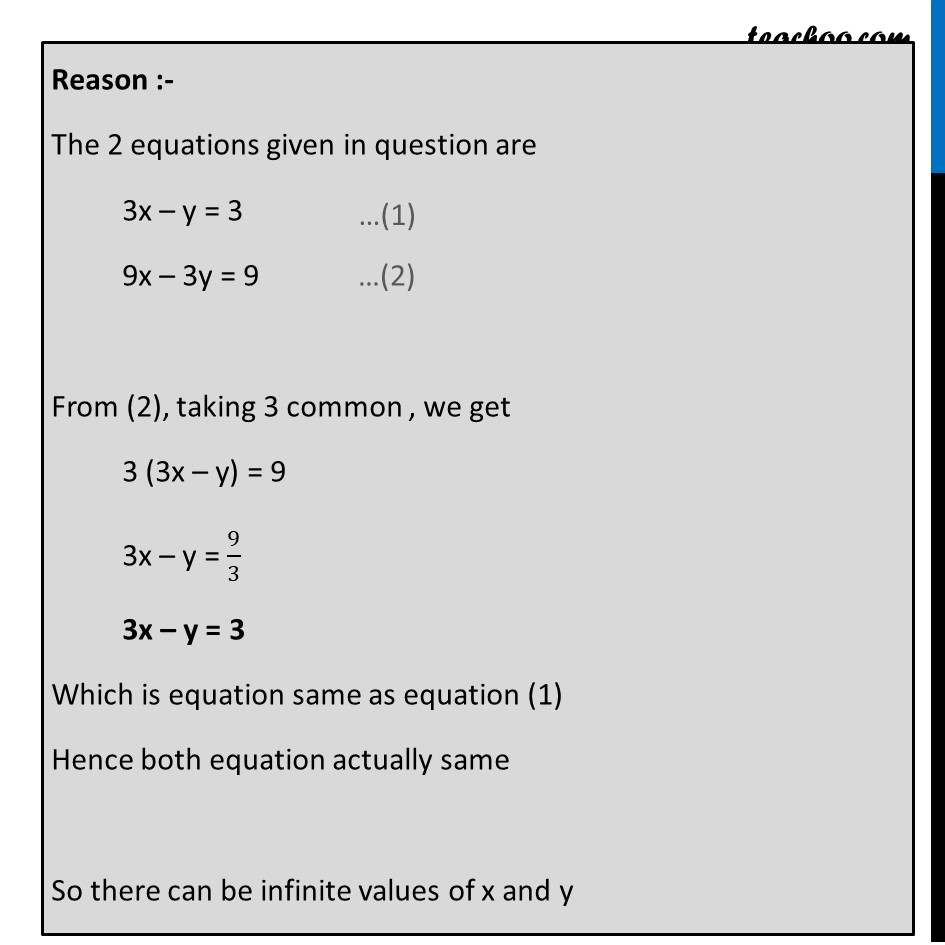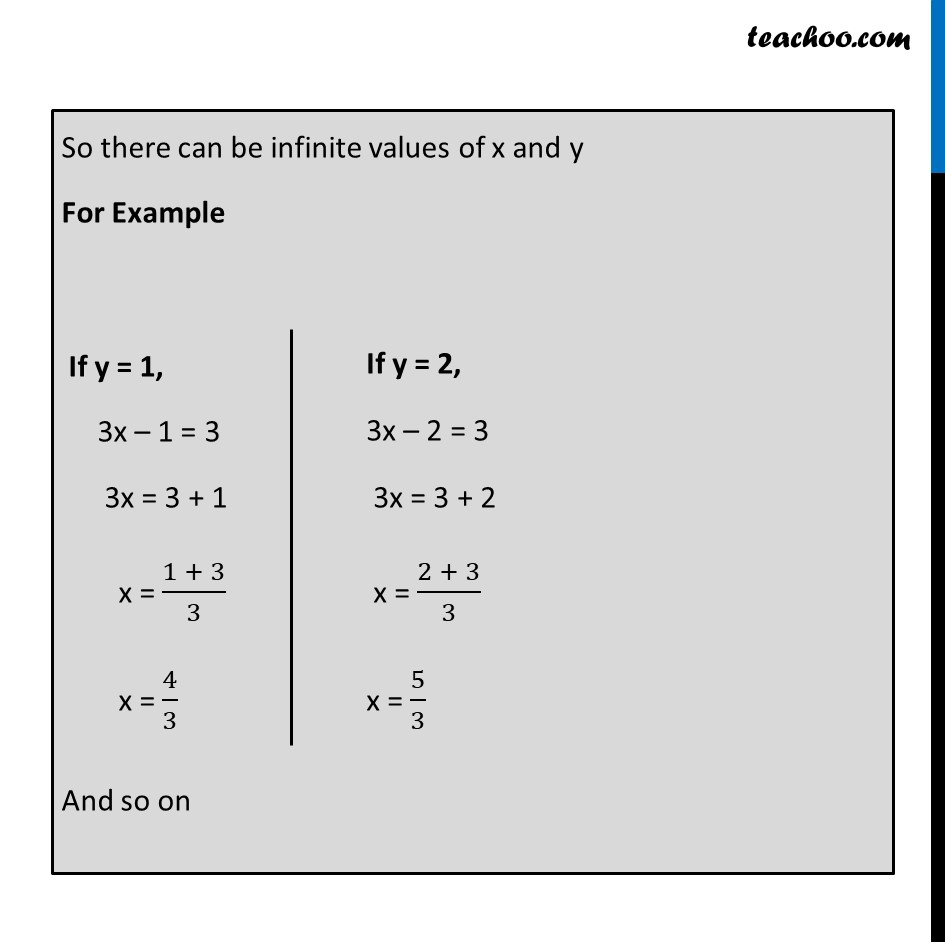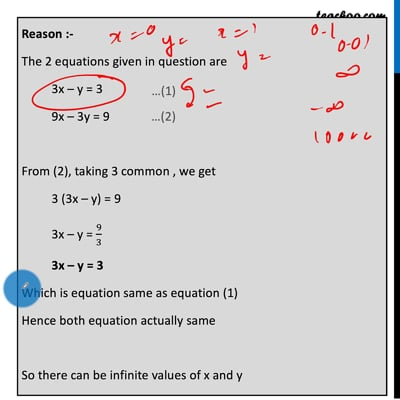This video is only available for Teachoo black users

Get live Maths 1-on-1 Classs - Class 6 to 12

### Transcript

Ex 3.3, 1 Solve the following pair of linear equations by the substitution method. (iii) 3x – y = 3 9x – 3y = 9 3x – y = 3 9x – 3y = 9 Solving (1) 3x – y = 3 3x = y + 3 x = (𝒚 + 𝟑)/𝟑 Putting value of x in (2) 9x – 3y = 9 9((𝑦 + 3)/3)−3𝑦=9 3(y + 3) – 3y = 9 3y + 9 – 3y = 9 3y – 3y + 9 = 9 0 + 9 = 9 9 = 9 The statement is true for all values of x So, there are infinitely many solutions Reason :- The 2 equations given in question are 3x – y = 3 9x – 3y = 9 From (2), taking 3 common , we get 3 (3x – y) = 9 3x – y = 9/3 3x – y = 3 Which is equation same as equation (1) Hence both equation actually same So there can be infinite values of x and y So there can be infinite values of x and y For Example And so on